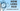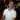# How long ago function

March 28, 2018

In the old days on the web, timestamps would just be a date; forum posts, blog posts, etc. would be just the date. Later on, we got comments in blog posts, and this would have the date and time. However, this wasn’t always very readable. In reality, people say how long ago something happened, rather than a date and time. “Oh, it was a few minutes ago” or “about 3 hours ago” rather than “it was on March 28th, 2018 at 3pm.” This idea of “how long ago” became popular in websites, mostly in the comments section. Check out YouTube, Stack Overflow, or other modern sites in the comments section and you’ll see that people posted comments some amount of “time ago.” Even on SME we do that in the comments section (thanks to the Disqus plug-in).

With this in mind, I thought it would be fun to create a VBA function that figures out “how long ago” something happened. I put together this function to share with you and I’ll explain how it works for those of you who really like to understand what’s going on.

Here’s what the output looks like (this screenshot was taken around 3/27/2018 11:49 PM):

Let’s dig into the function and how it works.

## How long ago function

Here is the VBA function:

``````Option Explicit

' Summary: Returns a friendly timestamp like "Just now" or "45 minutes ago"
' Param cell: The cell used for the function.
'             Should only be a single cell and should be a date with or without time.
Public Function HowLongAgo(ByRef cell As Range) As String
' Only allow dates and only allow single cells
If ((Not IsDate(cell)) Or cell.Cells.Count > 1) Then
HowLongAgo = vbNullString
Exit Function
End If

Dim amount As Long

amount = DateDiff("yyyy", cell, Now)

If (amount > 0) Then
HowLongAgo = GetHowLongAgoText(amount, "year")
Exit Function
End If

amount = DateDiff("m", cell, Now)

If (amount > 0) Then
HowLongAgo = GetHowLongAgoText(amount, "month")
Exit Function
End If

amount = DateDiff("d", cell, Now)

If (amount > 0) Then
HowLongAgo = GetHowLongAgoText(amount, "day")
Exit Function
End If

If (Not HasTimeValue(cell.value)) Then
HowLongAgo = "Today"
Exit Function
End If

amount = DateDiff("h", cell, Now)

If (amount > 0) Then
HowLongAgo = GetHowLongAgoText(amount, "hour")
Exit Function
End If

amount = DateDiff("n", cell, Now)

If (amount > 0) Then
HowLongAgo = GetHowLongAgoText(amount, "minute")
Exit Function
End If

amount = DateDiff("s", cell, Now)

If (amount >= 0) Then
HowLongAgo = GetHowLongAgoText(amount, "second")
Else
HowLongAgo = "Hasn't happened yet..."
End If
End Function

Private Function GetHowLongAgoText(ByVal amount As Long, ByRef unit As String) As String
Select Case amount
Case 0
GetHowLongAgoText = "Just now"
Case 1
GetHowLongAgoText = "1 " & unit & " ago"
Case 2
GetHowLongAgoText = "A couple " & unit & "s ago"
Case 3
GetHowLongAgoText = "A few " & unit & "s ago"
Case Else
GetHowLongAgoText = amount & " " & unit & "s ago"
End Select
End Function

Private Function HasTimeValue(ByRef value As String) As Boolean
HasTimeValue = InStr(1, value, ":")
End Function``````

Add this code to a module in your Excel workbook and you can reference it like this:

``=HowLongAgo(A2)``

Quick Tip

This function uses the Now function, which means that every time the cell this function lives in is recalculated, it will update the value if necessary.

Let’s break this down to understand how it works.

### Understanding the Function

We’ll start at the beginning. When we enter the function, we only want to use a cell with a date and only a single cell.

``````If ((Not IsDate(cell)) Or cell.Cells.Count > 1) Then
HowLongAgo = vbNullString
Exit Function
End If``````

If the cells is not a date, or if there is more than one cell passed in, we set the result to an empty string and return.

Now comes the fun part, we need to figure out how long ago the date happened. When thinking about this, I realized that it would be best to start off with the largest unit of time and if the result was 0, then move on to the next smaller unit. Then keep doing this until I find a non-zero number. Once found, that will be my units and we will work with that.

For example, if something happened 5 days ago, the logic goes like this:

• How many years ago was this? 0, ok move on to months
• How many months ago? 0, ok move on to days
• How many days ago? 5, perfect, we’ll use days

Imagine considering the opposite approach: starting with seconds, then moving up to minutes, hours, days, etc. The problem is where do you make the cutoff? If something happened 90 seconds ago, at what point do you move to minutes? At 6o seconds, right? OK, how about minutes? At 24, of course. Days? 30…

The problem with this approach is that you need way more logic to determine which unit to use. Whereas if you started with the largest unit, you can start at the largest and keep going until you find a non-zero answer. This makes the code very repeatable and less confusing.

Here’s a snippet of this logic in action:

``````Dim amount As Long

amount = DateDiff("yyyy", cell, Now)

If (amount > 0) Then
HowLongAgo = GetHowLongAgoText(amount, "year")
Exit Function
End If

amount = DateDiff("m", cell, Now)

If (amount > 0) Then
HowLongAgo = GetHowLongAgoText(amount, "month")
Exit Function
End If``````

The DateDiff() function is what we’ll be using for determining how long ago a date happened. Check out the MSDN docs for info on how to use this function. Basically, we first look at how many years have passed from the date and time that was passed in to Now (which is a VBA built-in function). If the difference is 0, we move on to the next smaller unit.

However, if the difference is greater than 0, then we ask another function `GetHowLongAgoText()` for the text we should give back to the cell.

``````Private Function GetHowLongAgoText(ByVal amount As Long, ByRef unit As String) As String
Select Case amount
Case 0
GetHowLongAgoText = "Just now"
Case 1
GetHowLongAgoText = "1 " & unit & " ago"
Case 2
GetHowLongAgoText = "A couple " & unit & "s ago"
Case 3
GetHowLongAgoText = "A few " & unit & "s ago"
Case Else
GetHowLongAgoText = amount & " " & unit & "s ago"
End Select
End Function``````

In this function, we pass in the amount of units that have passed, along with the unit we’re using (like “day” or “month”). We use a Select Case statement to figure out some of the smaller numbers.

Case Result Notes
`0` “Just now” This is only used for a date with a time that happened less than a minute ago.
`1` “1 `<unit>` ago” The reason I didn’t use “A `<unit>` ago” was because of “hour” as in “A hour ago” isn’t grammatically correct, so I chose to go with “1 `<unit>` ago” instead.
`2` “A couple of `<unit>`s ago” Works pretty well for all units.
`3` “A few `<unit>`s ago” Also works well for all units.
`Else` `<amount> <unit>`s ago” After 3, we just use numbers like “4 months ago.”

#### Handling when there is no time value

In the middle of the `HowLongAgo()` function, you’ll notice this line:

``````If (Not HasTimeValue(cell.value)) Then
HowLongAgo = "Today"
Exit Function
End If``````

We needed a way to determine if only a date value was placed in a cell (without any time value). So we call a function that simply looks at the cell to see if a colon is within the text (a clear indicator that there is a time component there) and if not, then the result should be `Today` since applying time would not make sense here.

However, if there is a time value, then we can move forward just like the previous items.

#### Handling future dates

Finally, we have this last portion of the function:

``````amount = DateDiff("s", cell, Now)

If (amount >= 0) Then
HowLongAgo = GetHowLongAgoText(amount, "second")
Else
HowLongAgo = "Hasn't happened yet..."
End If``````

Basically, if we’ve reached the point where we are looking at how many seconds has passed up to now and the date is in the future, then that means the `amount` will be a negative number. In this case, the event happens in the future and we respond accordingly with `Hasn't happened yet...`.## Conclusion

I hope this function was useful for you and if you have any comments, suggestions, or questions, please post them in the comments below!

Wow, you read the whole article! You know, people who make it this far are true learners. And clearly, you value learning. Would you like to learn more about Excel? Please consider supporting me by buying me a coffee (it takes a lot of coffee to write these articles!).Written by Joseph who loves teaching about Excel.